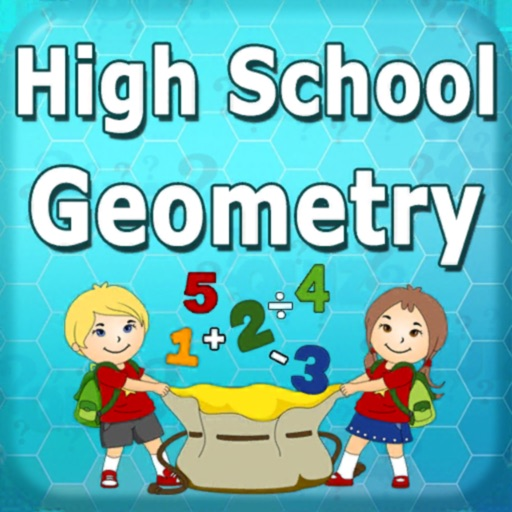High School Geometry Test Prep Practice math with unlimited questions in more than 200 Geometry Test Prep math skills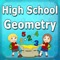High School Geometry Test Prep

by SoftSchools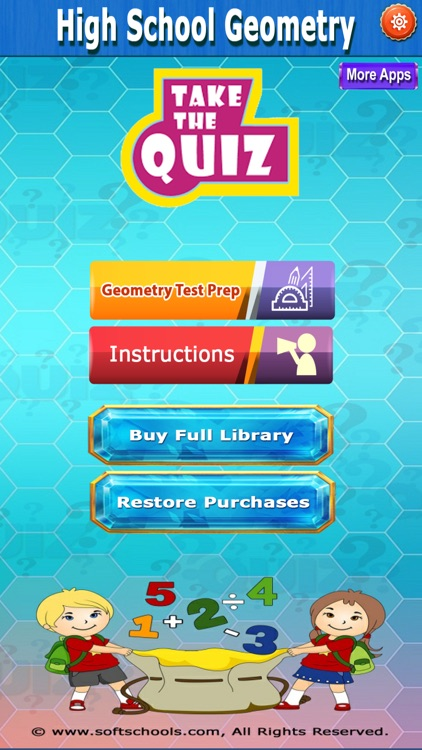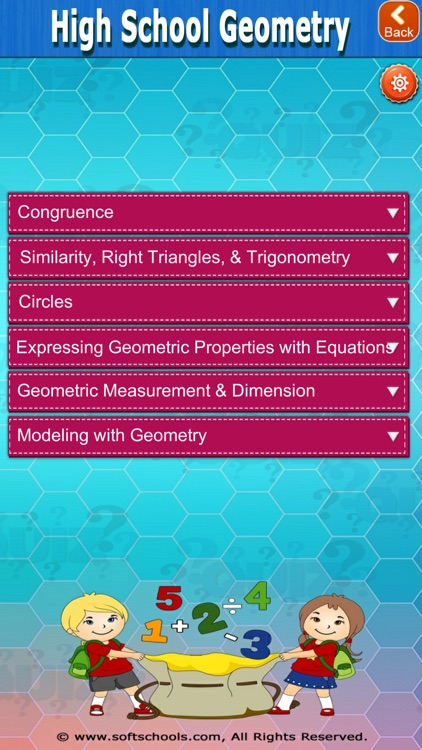High School Geometry Test Prep Practice math with unlimited questions in more than 200 Geometry Test Prep math skills.App Details

Version
1.01
Rating
NA
Size
100Mb
Genre
Education
Last updated
November 13, 2018
Release date
March 20, 2017

High School Geometry Test Prep is FREE but there are more add-ons

• \$4.99

High School Geometry Test Prep

App Screenshots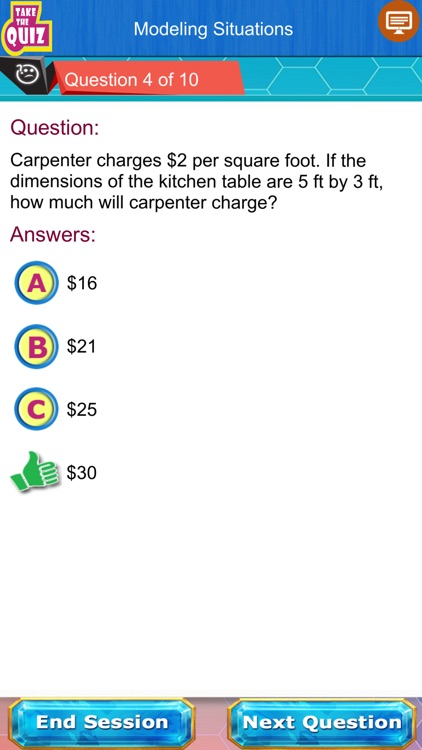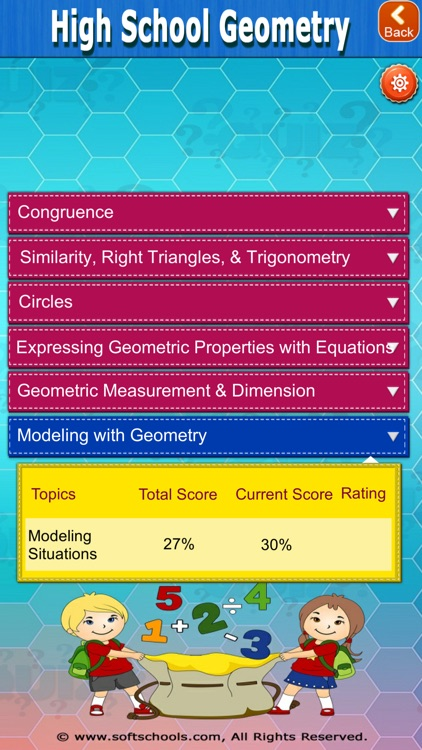App Store Description

High School Geometry Test Prep Practice math with unlimited questions in more than 200 Geometry Test Prep math skills.
The questions are specifically designed to match High School Geometry common core curriculum.
Each question has detailed explanation.
This High School Geometry test prep covers the following topics:

a) Congruence
1) Transformations
2) Rigid Motions
3) Geometric Theorems
4) Geometric Constructions

b) Similarity, Right triangles & Geometry
1) Trigonometry Triangles
2) Trigonometry Circles
3) Prove Theorems Similarity
4) Similarity Transformations

c) Circles
1) Circles
2) Areas of Sectors of Circles

d) Expressing Geometry Properties with equations
1) Geometric Description and the Equation for a Conic Section
2) Simple Geometric Theorems

e) Geometry Measurement and Dimension
1) Volume Formulas
2) Visualize Relationships between 2-D and 3-D Objects

f) Modelling with Geometry
1) Modeling Situations